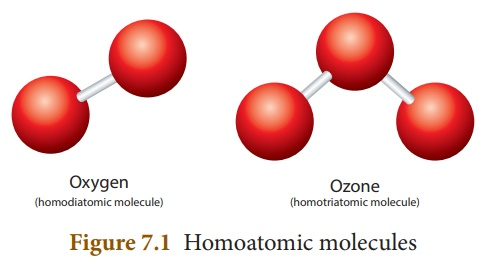Home | | Science 10th Std | Molecule and Molecular Mass

# Molecule and Molecular Mass

Except noble gases, atoms of most of the elements are found in the combined form with itself or atoms of other elements. It is called as a molecule. A molecule is a combination of two or more atoms held together by strong chemical forces of attraction, i.e. chemical bonds.

MOLECULE AND MOLECULAR MASS

Except noble gases, atoms of most of the elements are found in the combined form with itself or atoms of other elements. It is called as a molecule. A molecule is a combination of two or more atoms held together by strong chemical forces of attraction, i.e. chemical bonds.## 1. Classification of molecules

A molecule may contain atoms of the same element or may contain atoms of two or more elements joined in a fixed ratio, in accordance with the law of definite proportions. Thus, a ┬Łmolecule may be an element or a compound. If the molecule┬Ł is made of similar kind of ┬Łatoms, then it is called homoatomic molecule.

The molecule that consist of atoms of different elements┬Ł is called heteroatomic molecule. A compound is a heteroatomic molecule. The number of atoms present in the molecule is called its ŌĆśatomicityŌĆÖ.Let us consider oxygen. Oxygen gas exists in two allotropic forms: Oxygen (O2) and Ozone (O3). In oxygen molecule, there are two oxygen atoms. So its atomicity is two. Since both the atoms are similar, oxygen (O2) is a homodiatomic molecule. Other elements  that exist as diatomic molecules are hydrogen (H2), nitrogen (N2) and halogens: fluorine (F2), chlorine (Cl2), bromine (Br2) and iodine (I2).Ozone (O3) contains three oxygen atoms and hence it is called homotriatomic molecule. If a molecule contains more than three atoms, then it is called polyatomic molecule.

Conisder hydrogen chloride. It consists of two atoms, but of different elements, i.e. hydrogen and chlorine. So, its atomicity is two. It is a heterodiatomic molecule. Similarly, the water molecule contains two hydrogen atoms and one oxygen atom. So its atomicity is three. It is a heterotriatomic molecule.## 2.Relative Molecular Mass (RMM)

As the molecules are made of atoms, they also have their own mass. The mass of the molecule of an element or compound is measured in the C-12 scale and hence called relative molecular mass.

The Relative Molecular Mass of a molecule is the ratio between the mass of one molecule of the substance to 1/21th mass of an atom of Carbon -12.

The relative molecular mass is obtained by adding together the relative atomic masses of all the atoms present in a molecule.

## Calculation of relative molecular mass ŌĆō Solved examples:

Example 1: Relative molecular mass of sulphuric acid (H2SO4) is calculated as follows: Sulphuric acid conatins 2 atoms of hydrogen, 1 atom of sulphur and 4 atoms of oxygen.

Therefore, Relative molecular mass of sulphuric acid = (2 ├Ś mass of hydrogen) +  (1 ├Ś mass of sulphur) + (4 ├Ś mass of oxygen)

= (2 ├Ś 1) + (1 ├Ś 32) + (4 ├Ś 16)

= 98

i.e., one molecule of H2SO4 is 98 times as heavy as 1/12th of the mass of a carbon ŌĆō12.

Example 2: Relative molecular mass of water (H2O) is calculated as follows: A water molecule is made of 2 atoms of hydrogen and one atom of oxygen.

So, the relative molecular mass of water

= (2 ├Ś mass of hydrogen) + (1 ├Ś mass of  oxygen)

= (2 ├Ś 1) + (1 ├Ś 16)

= 18

i.e., one molecule of H2is 18 times as heavy as 1/12th of the mass of a carbon ŌĆō12.

Study Material, Lecturing Notes, Assignment, Reference, Wiki description explanation, brief detail
10th Science : Chapter 7 : Atoms and Molecules : Molecule and Molecular Mass |